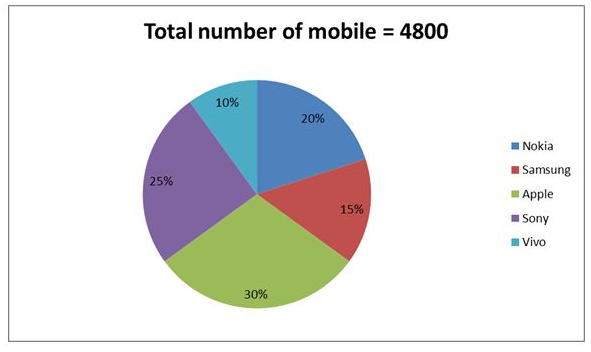# RRB Clerk Prelims Quantitative Aptitude (Day-15)

## Quantitative Aptitude Materials for RRB Clerk

Dear Aspirants, Our IBPS Guide team is providing new series of Quantitative Aptitude Questions for RRB Clerk 2020 Prelims so the aspirants can practice it on a daily basis. These questions are framed by our skilled experts after understanding your needs thoroughly. Aspirants can practice these new series questions daily to familiarize with the exact exam pattern and make your preparation effective.

Start Quiz

Ensure Your Ability Before the Exam Take IBPS RRB Clerk 2020 Prelims Free Mock Test

Approximation

Direction (1-2): What approximate value should come in the place of (?) in the following questions

1) √1850.184 * √169.169 – 139.915 – 250.239 = ?2

A) 13

B) 10

C) 19

D) 16

E) 21

2) 11.972 + 612.142 ÷ √289.144 – 28.01 =? * 4.901

A) 1

B) 4

C) 7

D) 10

E) 13

Direction (3-5): Following question contains two equations as I and II. You have to solve both equations and determine the relationship between them and give answer as,

a) x > y

b) x ≥ y

c) x = y or relationship can’t be determined.

d) x < y

e) x ≤ y

3)

I) x2– 17x + 42 = 0

II) y2– 16y + 28 = 0

4)

I) 2x2– 18x – 72 = 0

II) y2– 36y + 288 = 0

5)

I) 2x + y = -5

II) 3x + 3y = -9

Data interpretation

Direction (6-10): Study the following information carefully and answer the questions given below.

The given pie graph shows the number of mobiles in five different companies- Nokia, Samsung, Apple, Sony and Vivo.6) If 70% of the Nokia mobile is sold, find the number of unsold Nokia mobile?

A) 218

B) 267

C) 276

D) 288

E) None of these

7) The number of Samsung mobile is what percent of the number of Sony mobile?

A) 40%

B) 50%

C) 60%

D) 70%

E) None of these

8) What is the average number of Apple, Sony and Vivo mobiles together?

A) 1040

B) 1103

C) 1085

D) 1125

E) None of these

9) What is the ratio of the number of Nokia and Vivo mobile together to the number of apple mobile?

A) 2:1

B) 3:2

C) 4:3

D) 5:4

E) None of these

10) Ratio of the number of boys to girls in the class A is 4: 5 and the ratio of the number of boys to girls in class B is 3: 2. What is the ratio of the boys to girls in the school (both class A and B)?

A) 3: 2

B) 4: 1

C) 5: 3

D) Cannot be determined

E) None of these

Directions (1-2):

√1850.184 * √169.169 – 139.915 – 250.239 = ?2

√1849 * √169 – 140 – 250 = ?2

43 * 13 – 140 – 250 = ?2

? = 13

11.972 + 612.142 ÷ √289.144 – 28.01 =? * 4.901

12 + 612 ÷ √289 – 28 = ? * 5

12 + 36 – 28 = ? * 5

?=4

Directions (3-5):

x2 – 17x + 42 = 0

x2 – 14x – 3x + 42 = 0

x(x – 14) – 3(x – 14) = 0

(x – 3)(x – 14) = 0

x = 3, 14

y2 – 16y + 28 = 0

y2 – 14y – 2y + 28 = 0

y(y – 14) – 2(y – 14) = 0

(y – 2)(y – 14) = 0

y = 2, 14

Relationship between x and y cannot be established.

2x2 – 18x – 72 = 0

2x2 – 24x + 6x – 72 = 0

2x(x – 12) + 6(x – 12) = 0

(2x + 6)(x – 12) = 0

x = -3, 12

y2 – 36y + 288 = 0

y2 – 24y – 12y + 288 = 0

y(y – 24) – 12(y – 24) = 0

(y – 12)(y – 24) = 0

y = 12, 24

x ≤ y

2x + y = -5 ————(1)

3x + 3y = -9 ————–(2)

(1) * 3 – (2)

3x = -6

x = -2

y = -5 + 4 = -1

x < y

Directions (6-10):

Number of Nokia mobile = 20/100 * 4800 = 960

Number of unsold Nokia mobile = 960 * 30/100 = 288

Required ratio = 15/25 * 100 = 60%

Apple = 30/100 * 4800 = 1440

Sony = 25/100 * 4800 = 1200

Vivo = 10/100 * 4800 = 480

Average = (1440 + 1200 + 480)/3 = 1040

Required ratio = (20 + 10):30

= 1:1